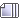###Author Topic: Grumpy's Algebraic therory  (Read 5888 times)

#### Kalphiter

Wrong! 0 1 2 3 4 5 is right! Why?

0+5=5
1+4=5
2+3=5
3+2=5
4+1=5
0+5=5

I meant all positive numbers!
1.1+3.9
1.01+3.99
1.001+3.999

And go on forever.

#### Deoxy99

Oh! I meant no fractions or decimals!

#### Deep2

Oh dear what shall we do!

#### Deoxy99

No in the problem!

#### Deoxy99

PROBLEM! 1/2+x=y

y=5/6

X cannot equal 1/2 1/4 1/6 1/8

or any of those with varying numbers in the first number.

#### Kalphiter

PROBLEM! 1/2+x=y

y=5/6

X cannot equal 1/2 1/4 1/6 1/8

or any of those with varying numbers in the first number.

x = 1 ÷ 3

#### Deoxy99

Wrong! I said no 1/6 2/6 3/6 4/6 5/6 6/6!

#### MtnDew

.3333333333333333333333333333 333333333333333333

#### Deoxy99

Okay...you are observing a box that looks like it is 75% off the building but not tilting! You run up to fix it! And now you see it moving! Why could that be?

#### fireball

Find the slope of the graph of y = (x-3)^1/2 when x = 2.

#### Deoxy99

Are you answering the problem? That problem is not algebra! It is a puzzle I made up!

#### Deep2

Are you answering the problem? That problem is not algebra! It is a puzzle I made up!

Algebra

noun

1. the branch of mathematics that deals with general statements of relations, utilizing letters and other symbols to represent specific sets of numbers, values, vectors, etc., in the description of such relations.

2. any of several algebraic systems, esp. a ring in which elements can be multiplied by real or complex numbers (linear algebra) as well as by other elements of the ring.

3. any special system of notation adapted to the study of a special system of relationship: algebra of classes.

No MINE!